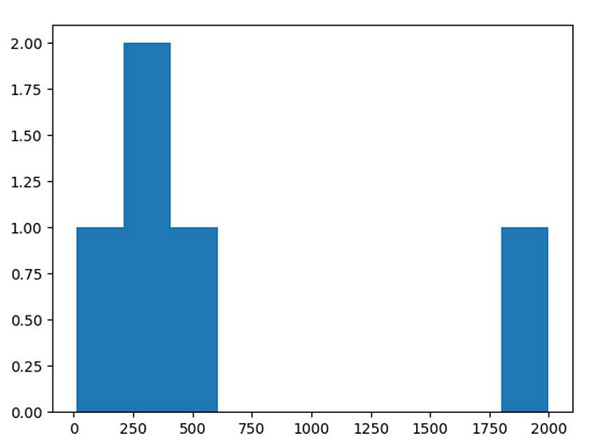# How to plot a histogram using Matplotlib in Python with a list of data?

To plot a histogram using Matplotlib, we can follow the steps given below −

• Make a list of numbers and assign it to a variable x.

• Use the plt.hist() method to plot a histogram.

• Compute and draw the histogram of *x*.

• We can pass n-Dimensional arrays in the hist argument also.

• To show the plotted figure, use the plt.show() method.

## Example

from matplotlib import pyplot as plt

x = [300, 400, 500, 2000, 10]

plt.hist(x, 10)

plt.show()

## Output